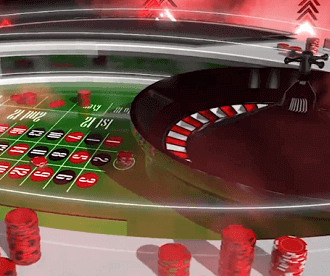# The Little-Known Tips For Odds and House Edges

In write ups about online games certain terms are repeated often. Some of these are probability, odds, house edges and payout ratios. Players do have a general idea of what these terms imply. For example they know that if a game has a higher house edge then they are more likely to lose money in that game. However not all players know the exact significance of these terms. One can get by without getting down to the nitty-gritty but it always helps to know the details.

The probability is the likelihood of an event occurring. In the European Roulette wheel there are 37 numbers, including the 0, and each of them are equally likely to be called. Therefore the probability of 13 being called is 1 out of 37. There are 18 red numbers and therefore the probability of a red number being called is 18 out of 37, which is marginally less than half. What this means is that if the game is fair and the wheel is spun a very large number of times then the number 13 will appear on an average once in every 37 spins.

Odds are the measure of how much the casino pays out if the player wins. Therefore odds are more commonly referred to as payouts. The odds for a single number bet in roulette are 35 to 1. This means that if the player wagers \$1 on the number 13 and 13 is called then the player will win \$35. The \$1 wagered by the player will also be returned to him. There are other methods of stating the odds as well and therefore the player must be careful not to mix them up.

House edge is a little more difficult to calculate. It is a measure of how much money the casino expects to make from each wager in the long run. Assume that a player wagers for 3,700 spins on the roulette wheel. That is a fairly good representation of the long term. Further assume that hewagers \$1 on the number 13 each time. Therefore the total amount the player wagers will be \$3,700. In the long run for every 37 spins the number 13 will be called once. Therefore in 3,700 spins it will be called 100 times. Each time the player will get \$35 in winnings and \$1 of his original wager, or \$36 in all. Hence in 100 wins he will get \$3,600. Therefore on his investment of \$3,700 the player expects to lose \$100 or the casino expects to make \$100. Therefore the percentage expectation that the casino has is 2.7% (100x\$100/\$3,700). This is the house edge.

The house edge is what the online casino gets. The average payout ratio is what the player gets. In the above example the player can expect to get \$3,600 out of every \$3,700 wagered. Therefore the average payout ratio will be 97.3% (100x\$3,600/\$3,700). The average payout ratio can be obtained by subtracting the house edge from 100.